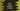# Java stream mapToInt explanation with examples## Java stream mapToInt:

mapToInt is used to get one integer stream from a different stream by applying a function to all members of a stream. This is an intermediate operation similar to map, filter and other intermediate operations.

### Syntax of mapToInt:

Below is the syntax of mapToInt method:

``IntStream mapToInt (ToIntFunction<? super T> mapper)``

It returns one IntStream by applying the mapper function to all elements of the stream.

In this post, I will show you different examples of mapToInt.

### Example 1: Using mapToInt to get the lengths of strings in a stream:

Let’s take a look at the below example:

``````import java.util.Arrays;
import java.util.List;

public class Example {
public static void main(String[] args){
List<String> givenList = Arrays.asList("one", "two", "three", "four");
givenList.stream().mapToInt(String::length).forEach(System.out::println);
}
}``````

In this example, we are creating one stream and using mapToInt to find the length of each string. Using forEach, we are printing the value of each item of the integer stream. It will give the below output:

``````3
3
5
4``````

### Example 2: Find the sum of lengths of all strings in a stream using mapToInt:

We can also find the sum of all values of the IntStream it returns:

``````import java.util.Arrays;
import java.util.List;

public class Example {
public static void main(String[] args){
List<String> givenList = Arrays.asList("one", "two", "three", "four");
int sum = givenList.stream().mapToInt(String::length).sum();
System.out.println(sum);
}
}``````

It will print 15.

### Example 3: Using filter with mapToInt:

We can use filter with mapToInt as like below:

``````import java.util.Arrays;
import java.util.List;

public class Example {
public static void main(String[] args){
List<String> givenList = Arrays.asList("one", "two", "three", "four");
int sum = givenList.stream().mapToInt(String::length).filter(value -> value % 2 == 0).sum();
System.out.println(sum);
}
}``````

In this example, we are filtering out the values of lengths of the strings and finding the sum of all lengths of the strings those are even. It will print 4.